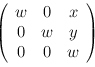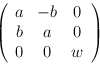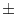Next: Point Generators ( point Up: Basic Data Types for Previous: Geometry Algorithms ( geo_alg   Contents   Index

# Transformation ( TRANSFORM )

Definition

There are three instantiations of TRANSFORM: transform (floating point kernel), rat_transform (rational kernel) and real_transform (real kernel). The respective header file name corresponds to the type name (with .h'' appended).

An instance T of type TRANSFORM is an affine transformation of two-dimensional space. It is given by a 3 x 3 matrix T with T2, 0 = T2, 1 = 0 and T2, 20 and maps the point p with homogeneous coordinate vector (px, py, pw) to the point T*p.

A matrix of the formrealizes an translation by the vector (x/w, y/w) and a matrix of the formwhere a2 + b2 = w2 realizes a rotation by the angleabout the origin, where cos= a/w and sin= b/w. Rotations are in counter-clockwise direction.

#include < LEDA/geo/generic/TRANSFORM.h >

Creation

 TRANSFORM T creates a variable introduces a variable T of type TRANSFORM. T is initialized with the identity transformation. TRANSFORM T(const INT_MATRIX t) introduces a variable T of type TRANSFORM. T is initialized with the matrix t. Precondition t is a 3 x 3 matrix with t2, 0 = t2, 1 = 0 and t2, 20.

Operations

 INT_MATRIX T.T_matrix() returns the transformation matrix void T.simplify() The operation has no effect for transform. For rat_transform let g be the ggT of all matrix entries. Cancels out g. RAT_TYPE T.norm() returns the norm of the transformation TRANSFORM T(const TRANSFORM& T1) returns the transformation ToT1. POINT T(const POINT& p) returns T(p). VECTOR T(const VECTOR& v) returns T(v). SEGMENT T(const SEGMENT& s) returns T(s). LINE T(const LINE& l) returns T(l ). RAY T(const RAY& r) returns T(r). CIRCLE T(const CIRCLE& C) returns T(C). POLYGON T(const POLYGON& P) returns T(P). GEN_POLYGON T(const GEN_POLYGON& P) returns T(P).

Non-member Functions

In any of the function below a point can be specified to the origin by replacing it by an anonymous object of type POINT, e.g., rotation90(POINT()) will generate a rotation about the origin.

 TRANSFORM translation(const INT_TYPE& dx, const INT_TYPE& dy, const INT_TYPE& dw) returns the translation by the vector (dx/dw, dy/dw). TRANSFORM translation(const RAT_TYPE& dx, const RAT_TYPE& dy) returns the translation by the vector (dx, dy). TRANSFORM translation(const VECTOR& v) returns the translation by the vector v. TRANSFORM rotation(const POINT& q, double alpha, double eps) returns the rotation about q by an angle alphaeps. TRANSFORM rotation90(const POINT& q) returns the rotation about q by an angle of 90 degrees. TRANSFORM reflection(const POINT& q, const POINT& r) returns the reflection across the straight line passing through q and r. TRANSFORM reflection(const POINT& q) returns the reflection across point q.Next: Point Generators ( point Up: Basic Data Types for Previous: Geometry Algorithms ( geo_alg   Contents   Index